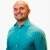# SAT Prep How to multiply two algebraic expressions by using the properties of exponentsThis lesson will look at an example problem involving exponents. This lesson will give an explanation of how to multiply two algebraic expressions by using the properties of exponents with a step by step look to... This lesson will look at an example problem involving exponents. This lesson will give an explanation of how to multiply two algebraic expressions by using the properties of exponents with a step by step look to better explain the concept.
More... Collapse
7 Views